1. /
2. CBSE
3. /
4. Class 06
5. /
6. Mathematics
7. /
8. CBSE Class 6 Maths...

# CBSE Class 6 Maths Practical Geometry Chapter 14 Extra Questions### myCBSEguide App

Download the app to get CBSE Sample Papers 2023-24, NCERT Solutions (Revised), Most Important Questions, Previous Year Question Bank, Mock Tests, and Detailed Notes.

CBSE Class 6 Maths Practical Geometry Chapter 14 Extra Questions. myCBSEguide has just released Chapter Wise Question Answers for class 6 Maths. There chapter wise Practice Questions with complete solutions are available for download in myCBSEguide website and mobile app. These Extra Questions with solution are prepared by our team of expert teachers who are teaching grade in CBSE schools for years. There are around 4-5 set of solved Mathematics Extra questions from each and every chapter. The students will not miss any concept in these Chapter wise question that are specially designed to tackle Exam. We have taken care of every single concept given in CBSE Class 6 Mathematics syllabus and questions are framed as per the latest marking scheme and blue print issued by CBSE for Class 6.

CBSE Class 6 Maths Extra Questions

## Important Questions for Class 6 Chapter 14 Mathematics

Ch-14 Practical Geometry

1. Angles of set squares are 45, 90 and ____.
1. 60
2. 75
3. 30
4. 90
2. A ________ is the longest chord of a circle.
1. diameter
3. None of these
4. chord
3. If the radius of a circle is 8.5 cm, then the diameter of the circle is ____.
1. None of these
2. 17 cm
3. 12 cm
4. 8.5 cm
4. If the radius of a circle is 3 cm, then the diameter of the circle is ____.
1. 1.5 cm
2. None of these
3. 6 cm
4. 3 cm
5. If the radius of a circle is 5.5 cm, then the diameter of the circle is ____.
1. 11 cm
2. 5.5 cm
3. 12 cm
4. ​​​​​​None of these
6. Match the following:

Column AColumn B
(a) The line which divides a line segment into two equal halves and perpendicular to it is called(p) perpendicular lines
(b) The line which divides an angle into two equal angles is called(q) parallel lines
(c) The lines which intersect each other at 90o are called(r) perpendicular bisector
(d) Two lines which are parallel to the same line are called(s) angle bisector
7. Fill in the blanks:

1. The image of points A and B in the line l are P and Q respectively then {tex}\overline {PQ} {/tex} = ________.
2. To bisect a line segment of length 5cm, the opening of the compass should be more than half of ________.
3. If an angle of measure 90° is bisected twice the angle so obtained measures _________.
4. In an isosceles ∆PQR, the bisector of ∠Q and ∠R meet at O. If ∠QOR = 140°, then ∠P = ______.
8. State whether the following statements are true or false:

1. Two line segments are compared in terms of their lengths.
2. When a ray makes one complete rotation, the measure of angle formed is 90°.
3. With the help of compasses we can draw 80°.
4. To construct an angle of {tex}37\frac{1}{2}^\circ {/tex}, we can bisect 75°.
9. If an angle of 110o is bisected, find the measure of each angle formed.
10. Draw two line segments which are perpendicular to each other using set squares.
11. Construct an angle of 60° using compass and ruler.
12. Construct {tex}\overline {PQ} {/tex} of length 6cm. From this cut off {tex}\overline {PR} {/tex} of length {tex}\overline {PR} {/tex} of length 4.5cm. Measure {tex}\overline {QR} {/tex}.
13. Draw any circle and mark points A, B and C such that:

1. A is on the circle.
2. B is the interior of the circle.
3. C is the exterior of the circle.
14. With {tex}\overline{\mathrm{PQ}} {/tex} of length 6.1cm as diameter draw a circle.
15. Draw a circle with centre C and radius 3.4cm. Draw any chord {tex}\overline{AB}{/tex}. Construct the perpendicular bisector of {tex}\overline{AB}{/tex} and examine, if it passes through C.

Ch-14 Practical Geometry

1. (b) 75
Explanation: set squares come in two forms, both right triangles, it has 90-45-90 degree angles. Therefore the third angle here will be 90 degree
1. diameter
Explanation: A diameter joins two points on the circle and so it is a chord. The diameter also passes through the centre of the circle and is the longest chord of the circle.
1. 17 cm
Explanation: Diameter is twice the radius.
Diameter = radius x 2 = 8.5 x 2 = 17 cm
1. 6 cm
Explanation: Diameter is twice the radius.
Diameter = radius x 2 = 3 x 2 = 6 cm
1. 11 cm
Explanation: Diameter is twice the radius.
Diameter = radius x 2 = 5.5 x 2 = 11 cm
1. {tex} \to {/tex} r
2. {tex} \to {/tex} s
3. {tex} \to {/tex} p
4. {tex} \to {/tex} q
1. {tex}\overline {AB} {/tex}
2. 5cm
3. {tex}22\frac{1}{2}^\circ {/tex}
4. 100o
1. True
2. False
3. False
4. True
1. If an angle of 110o is bisected (divided into two equal parts), then each angle would be {tex}\frac{{110^\circ }}{2} = 55^\circ {/tex}.
2.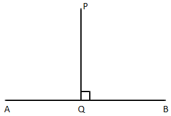3.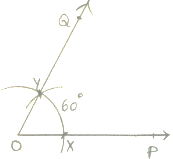Steps of Construction:
1. Draw a ray {tex}\overrightarrow {OP} {/tex}.
2. With ‘O’ as centre and any radius, draw an arc. Cutting {tex}\overrightarrow {OP} {/tex} at x.
3. With x as centre and the same radius, draw another arc intersecting the first arc at y
4. Join O, Y and produce it to Q.
Hence, {tex}\angle POQ = 60^\circ {/tex} is the required angle.
4.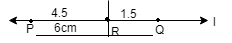steps:-

1. Place the zero mark of the ruler at ‘P’.
2. Mark a point ‘Q’ at a distance of 6cm from ‘P’.
3. Again mark a point ‘R’ at a distance of 4.5cm from ‘P’.
4. Hence by measuring {tex}\overline {QR} {/tex} we find {tex}\overline {QR} {/tex} = 6 – 4.5 = 1.5cm.
1.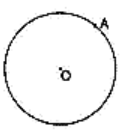2.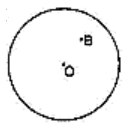3.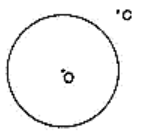5.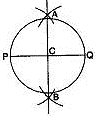1. Draw a line segment {tex}\overline{\mathrm{PQ}} {/tex} of length 6.1cm.
2. With P as centre, using compasses, draw an arc. The radius of this arc should be more than half of the length of {tex}\overline{\mathrm{PQ}} {/tex}.
3. With the same radius and with Q as centre, draw another arc using compasses. Let it cut the previous arcs at A and B.
4. Join {tex}\overline{A B}{/tex}. It cuts {tex}\overline{\mathrm{PQ}} {/tex} at C. Then {tex}\overline{A B}{/tex} is the perpendicular bisector of the line segment {tex}\overline{\mathrm{PQ}} {/tex}.
5. Place the pointer of the compasses at C and open the pencil upto P.
6. Turn the compasses slowly to draw the circle.
6.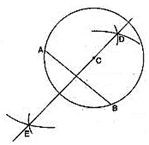1. Draw a point with a sharp pencil and mark it as C.
2. Open the compasses for the required radius 3.4cm, by putting the pointer on 0 and opening the pencil upto 3.4cm.
3. Place the pointer of the compasses at C.
4. Turn the compasses slowly to draw the circle.
5. Draw any chord {tex}\overline{AB}{/tex} of this circle.
6. With A as centre, using compasses, draw an arc. The radius of this arc should be more than half of the length of {tex}\overline{AB}{/tex}.
7. With the same radius and with B as centre, draw another arc using compasses. Let it cut the previous arcs at D and E.
8. Join {tex}\overline {DE}{/tex}. Then {tex}\overline {DE}{/tex} is the perpendicular bisector of the line segment {tex}\overline{AB}{/tex}. On examinating, we find that it passes through C.

## Chapter Wise Extra Questions for Class 6 MathematicsTest Generator

Create question paper PDF and online tests with your own name & logo in minutes.myCBSEguide

Question Bank, Mock Tests, Exam Papers, NCERT Solutions, Sample Papers, Notes

### 2 thoughts on “CBSE Class 6 Maths Practical Geometry Chapter 14 Extra Questions”

1. very nice and easy qusetions ………..

2. Great worksheet but it could be better with answers